Rg-Calculator for Polygonal Prisms and Cylinders

Enter either the edge length (a), circum-radius (a) or in-radius (a) and height (h) of a solid prism with its polygonal base (cross-section) being equilaterally triangular (trigonal), quadratic (tetragonal), pentagonal, hexagonal and octagonal, respectively, and for comparison also the radius (=a) and height (h) of a circular cylinder. Their radius of gyration (Rg) and their volume will be calculated. Units are arbitrary.

 Parameter Triangular Quadratic Pentagonal Hexagonal Octagonal Cylinder Shape of polygonal prism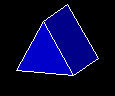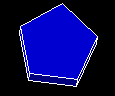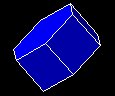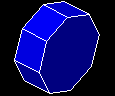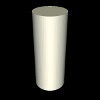Volume RgRg-Calculator for Polygonal Pyramids and Cones

Enter either the edge length (a), circum-radius (a) or in-radius (a) and height (h) of a solid pyramid and bi-pyramid with its polygonal base (cross-section) being equilaterally triangular (trigonal), quadratic (tetragonal), pentagonal, hexagonal and octagonal, respectively, and for comparison also the radius (=a) and height (h) in the z-direction (axis of revolution) of a circular (bi-)cone. Their radius of gyration (Rg) and their volume will be calculated for the mono- and bi-shape, respectively. Units are arbitrary.

 Parameter Triangular Quadratic Pentagonal Hexagonal Octagonal Cone Shape of polygonal bi-pyramid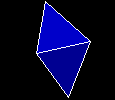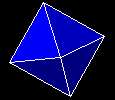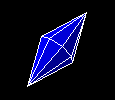Volume Rg (mono) Rg (bi)Universal Rg-Calculation for any solid Prism, (Bi-)Pyramid and Torus with an n-polygonal base

Enter the value n (>2) of the n-polygon and then either its edge length (a) or its circum-radius (a) or its in-radius (a). The radius of gyration (Rg) and volume of a prism, pyramid, bi-pyramid, double-pyramid and torus with the respective n-polygonal cross-section and with the height (h) will be calculated. In case of a torus, (h) is the central radius (=radius from the torus-center to the polygon's centroid). Units are arbitrary.

n : a : h : a = edge-length a = circum-radius a = in-radius

 Parameter Prism Pyramid Bi-Pyramid Double-Pyramid Torus Volume RgUniversal Rg-Calculation for any solid (Bi-)Pyramid with a triangular, rectangular or rhombic base

Enter the semi-axes ra, rb and height (h) of a pyramid with a triangular/rectangular/rhombic base. (In case of a triangular base ra and rb are the height and base of the isosceles triangle with edge lengths a,a,rb). The radius of gyration (Rg) and volume of the (bi-/double-)pyramid will be calculated. Units are arbitrary.

ra : rb : h :

 Parameter triangular rectangular rhombic Volume Rg (semi-) Rg bi- Rg double-Universal Rg-Calculation for any solid Cone, Paraboloid and Ellipsoid with a circular/elliptical cross-section

Enter the semi-axes ra, rb and height (h) of a cone. The radius of gyration (Rg) and volume of the (bi-/double-)cone, (bi-/double-)paraboloid, (semi-)ellipsoids will be calculated. Units are arbitrary.

ra : rb : h :

 Parameter Cone Paraboloid Ellipsoid Volume Rg (semi-) Rg bi- Rg double-Universal Rg-Calculation for any solid Cylinder with a (semi-)elliptical cross-section or for any solid Prism with a rectangular/triangular/rhombic base

Enter the semi-axes ra and rb of an ellipse, rectangle, rhombus and the height (h) of the resulting elliptical cylinder, rectangular prism, rhombic prism. The radius of gyration (Rg) and Volume (V) of the cylinder and prism will be calculated. For comparison also Rg of one quarter (Rg 1/4) and one half (Rg 1/2) of the geometric shapes will be given. The cuts (quarter 1/4, half 1/2) are symmetrically and centered through the cross-section or base. In case of cuts through the rhombic base, the resulting shape of the base is a right (1/4 cut) or isosceles (1/2 cut) triangle. Units are arbitrary.

ra : rb : h :

 Parameter (semi)-elliptical (triangular)/rhombic rectangular Volume (1/1) Rg (1/4) Rg (1/2) Rg (1/1) Rg (-1/-1)Universal Rg-Calculation for any solid Torus with an elliptical, rectangular or rhombic cross-section

Enter the semi-axes ra and rb of the elliptical, rectangular or rhombic cross-section of the torus with the central radius R. The radius of gyration (Rg) and Volume (V) of the torus will be calculated. Units are arbitrary.

ra : rb : R :

 Parameter elliptical rectangular rhombic Volume RgAuthor: M.Kriechbaum, TU-Graz (2013), e-mail: manfred.kriechbaum@tugraz.at